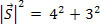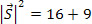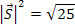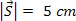Mechanics

# Unit vectors

We know that in Physics there are some quantities that need the identification of their intensity (a number followed by a unit of measurement) and their spatial orientation (direction and direction), in order to be well characterized. Such quantities, in Physics, are called vector quantities .

Associating to the x axis a vector, of unit modulus, that presents the same orientation of the axis, the component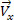can, therefore, be written in the form: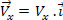Where Vx is the real number (positive, negative or null) called the projection of the vector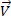on the x axis. If the componenthas the same direction as the x axis, the projection of V x will be a positive number; if it has the opposite direction, V x will be negative. Ifit is perpendicular to the x axis, its Vx projection will be zero.

Analogously, associating to the y-axis a vector, with a unit module, with the same orientation as the axis, the component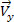can thus be written in the form: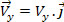Where Vy is the real number (positive, negative or null) called the projection of the vectoron the y-axis. If the componenthas the same orientation as the y-axis, the Vy projection will be a positive number; if it has the opposite direction, Vy will be negative. Ifit is perpendicular to the y-axis, its Vy projection will be zero.

Resolution

From the figure, we get the following data: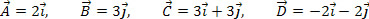So, the result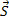is given by: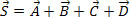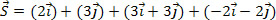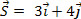Since the modules ofandare equal to 1, we have: